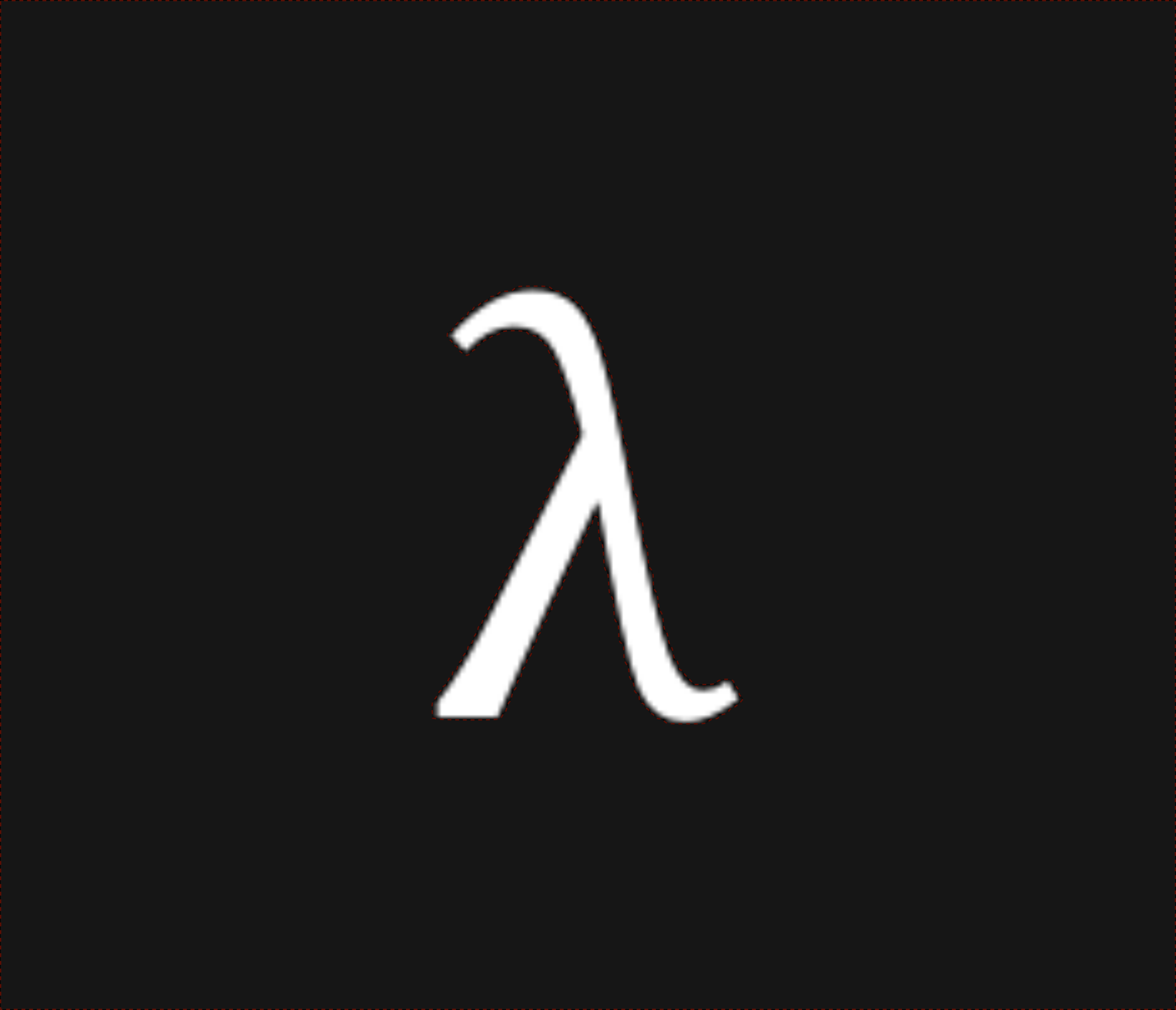nixpulvis

## Achilles' Tent Notes #10 written by Nathan Lilienthal on October 28, 2019.

### Typing Notes

• Oxide doesn’t have traits, or associated types
• Proper syntax for oblivious array types: obliv [τ; N], cases enumerated below:

Details:

• obliv [obliv τ; N]⋮Index<obliv usize> An oblivious array with oblivious data and oblivious access is an ORAM
• obliv [obliv τ; N]⋮Index<usize> An oblivious array with oblivious data and plain access
• obliv [τ; N]⋮Index<obliv usize> An oblivious array with plain data and oblivious access is comparable to a PIR, as it could simply reveal the whole array
• obliv [τ; N]⋮Index<usize> An oblivious array with plain data and plain access only adds a secure mapping of indices to logical memory addresses. Logical to physical mappings are still present in the face of compiled Rust
• [obliv τ; N]⋮Index<usize> is just a normal ol’ array of obliv τ types
• [τ; N] ⋮Index<obliv usize> is not implemented, and should be a type error
• obliv (), obliv (T), obliv (T, U), and so on…
• obliv (T) should not be equivalent to obliv T
• obliv (T, U) = (obliv T, obliv U), simply structural
• Rust’s struct ($$S::\langle\barρ,\barτ\rangle$$) are like tuples, so they should be consistent
• obliv obliv τ
• Supports “MPC in the head” nested protocols
• Might require careful consideration when writing typing rules with promotions
• conceal(τ) and reveal(τ) where τ: σ → ρ
• Initial thought is that it doesn’t make sense to allow this, because function bodies must be public information in general, more on this below
• Oxide doesn’t currently have enum or match
• match should be able to be represented as if, with an understanding of some patterns
• Enums are “tagged”, sum types
• We could start small and only allow matching for enums, avoiding the broader class of “patterns”

### Redex Model

• Started a redex model (define-language obliv)
• Thinking it should be (define-union-language ...)
• Example typing judgment:
[(types Γ e_1 (obliv bool))
(types Γ e_2 (obliv t))
(types Γ e_3 (obliv t))
-------------------------------------------- obliv-if
(types Γ (obliv-if e_1 e_2 e_3) (obliv t))])

• Example reduction rule:
(--> (in-hole E (obliv-if v_1 (λ (x : t) e_2)
(λ (x : t) e_3)))
(in-hole E (λ (x : t) (obliv-if v_1 e_2 e_3)))
"obliv-if-λ")


#### Public Promotion & Wire Types

• How can I write promotion rules like the following:
[(types Γ e t)
(side-condition (public? t))
---------------------------- promotion?
(types Γ e (obliv t))]

• New typing forms for public and obliv wire types
[t ::=
num
bool
(→ t t)
; ----------
(<public> t)
(<obliv> t)]

• (conceal (+ 1 2)) gets you (<obliv> 3)
• 1 used in place of a wire can be public promoted to (<public> 1)

Details:

• Public predicate and public values
(mux #t 2 3)
; -promotion>
(mux (<public> #t) (<public> 2) (<public> 3))
; -public-mux>
(<public> 2)
:
(<public> num)

• Public predicate and obliv values
(mux #f (conceal 2) (conceal 3))
; -promotion>
(mux (<public> #f) (conceal 2) (conceal 3))
; -conceals>
(mux (<public> #f) (<obliv> 2) (<obliv> 3))
; -public-mux>
(<obliv> 3)
:
(<obliv> num)

• obliv conditional predicate
(mux (conceal x) 1 2)
; -promotion>
(mux (<obliv> x) (<public> 1) (<public> 2))
:
(<obliv> num)

• (<public> (→ t t)) is the promotion of any function
• (<obliv> (→ t t)) could be the result type of something like (mux p (λ (x : num) x) (λ (x : num) 0)) since which function’s value is used will be unknown to an observer
• We may want the ability to send/recv (<public> v) at run-time